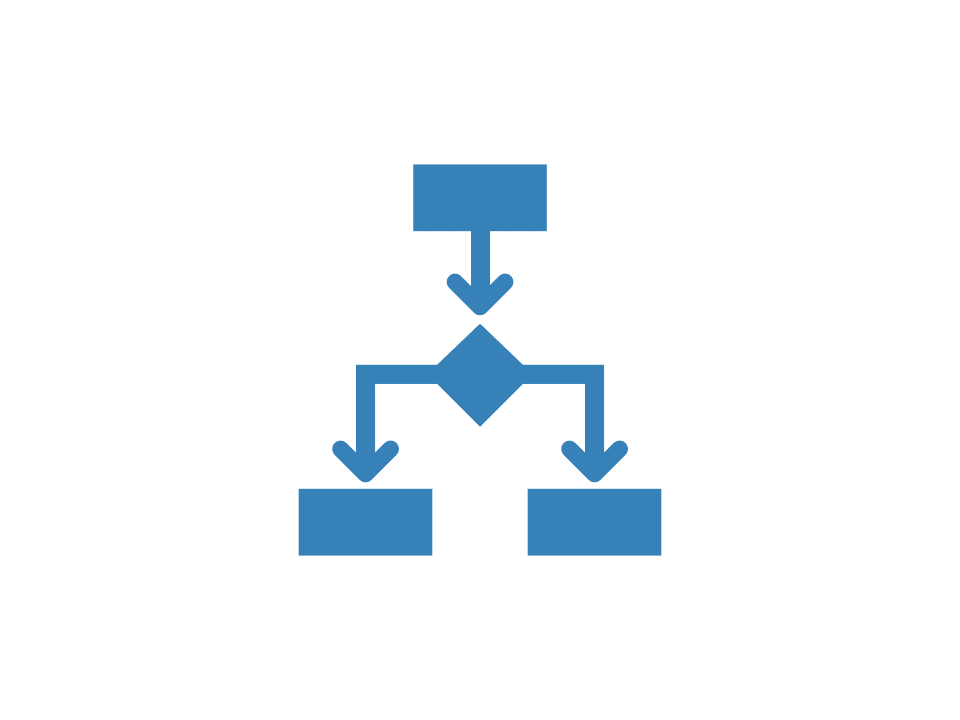# Frequency maps

library(bupaverse)

A process map of a log can be created using process_map(). A process map is a directly-follows graph, where each distinct activity is represented by a node, and each directly-follows relationship between activities is shown by directed edges, i.e. arrows between the nodes.

Below we explain how process maps can be used to show frequent (and infrequent) flows in the process. You can also use them to visualize performance or more advanced analyses.

Six different flavors of frequency process map can be considered:

• absolute frequency
• Nodes: The absolute number of activity instance executions
• Flows: The absolute number of times source and target activities were executed directly following each other.
• absolute-case frequency
• Nodes: The absolute number of cases in which the activity was executed
• Flows: The absolute number of cases in which source and target activity were executed directly following each other.
• relative frequency
• Nodes: The proportion of all activity instances that had this type
• Flows: The proportion of source executions directly followed by target executions.
• relative-case frequency
• Nodes: The proportion of cases in which the activity was executed
• Flows: The proportion of cases in which source and target activities were executed directly following each other.
• relative-antecedent frequency (= relative)
• Nodes: The proportion of all activity instances that had this type
• Flows: The proportion of source executions directly followed by target executions.
• relative-consequent frequency
• Nodes: The proportion of all activity instances that had this type
• Flows: The proportion of target executions that was preceded by an execution of source.

Each of these flavors can be configured by passing type = frequency() to process_map(), and additionally specifying the type of frequency() (ex. “absolute”, “absolute-case”, etc.)

In the examples below, we will use a slightly filtered versions of the traffic_fines data set, which contains 95% of the cases that have the most frequent traces.

tmp <- traffic_fines %>%
filter_trace_frequency(percentage = 0.95)

## Absolute

Below you can see the absolute frequency map. Let’s focus on the Payment activity. We see it was executed 4686 times. It was followed by another payment 250 times, while it was also the end of the case in 4436 times.

tmp %>%
process_map(frequency("absolute"))

Note that this is the default process map configuration, and is thus equivalent to the following.

tmp %>%
process_map()

## Absolute case

Looking at the absolute-case process map below, we see that the Payment activity is only executed in 4436 cases. This number is lower than the total number of executions seen above because of the self-loop on the activity.

tmp %>%
process_map(frequency("absolute-case"))

## Relative

In relative terms, Payment represents 14.51% of the total activity instances. We can furthermore see that in 94.66% of cases it occurred, it was the end of the case. In the other 5.34% of cases, it was followed by another Payment.

tmp %>%
process_map(frequency("relative"))

## Relative case

Below, we see that Payment occurred in 46.24% of cases. In 2.5% of cases, a Payment activity was followed by another Payment.

tmp %>%
process_map(frequency("relative-case"))

## Relative antecedence

See relative. Relative-antecedence is included as an option in symmetry with relative-consequent.

## Relative consequent

Finally, the relative-consequent map shows us what happens before activities. With respect to Payment, we can see that it was preceded by:

• Create Fine (73.15%)
tmp %>%
process_map(frequency("relative-consequent"))